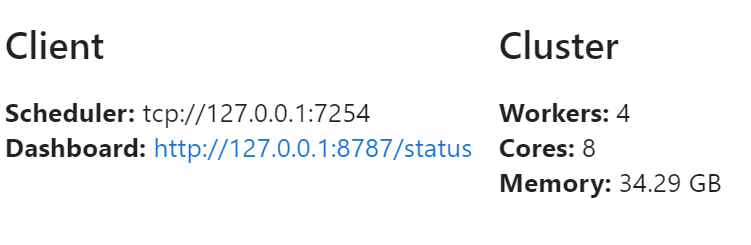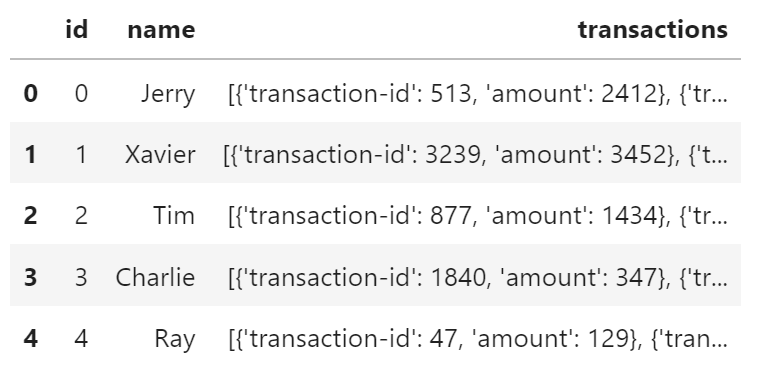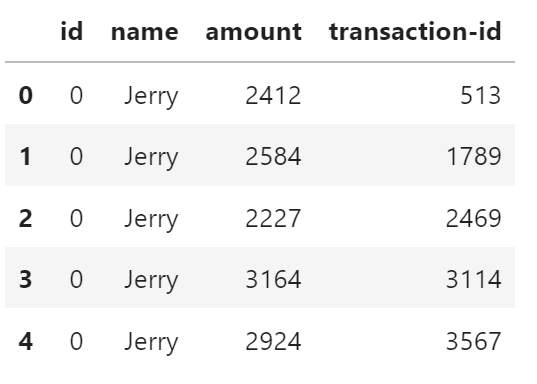Dask-bag 擅长处理可以表示为任意输入序列的数据。 我们将其称为“杂乱”数据，因为它可能包含复杂的嵌套结构、缺失字段、数据类型的混合等。 函数式编程风格非常适合标准 Python 迭代，例如可以在 itertools 模块中找到。

dask.bag = map, filter, toolz + parallel execution


## 创建数据

%run prep.py -d accounts


## 设置

from dask.distributed import Client

client = Client(n_workers=4)
client## 创建

import dask.bag as db


b = db.from_sequence(
[1, 2, 3, 4, 5, 6, 7, 8, 9, 10],
npartitions=2
)
b.take(3)

(1, 2, 3)


from pathlib import Path
b.take(1)

('{"id": 0, "name": "Jerry", "transactions": [{"transaction-id": 513, "amount": 2412}, ...)


import sources
sources.bag_url

's3://dask-data/nyc-taxi/2015/yellow_tripdata_2015-01.csv'


b = db.read_text(
sources.bag_url,
storage_options={"anon": True}
)
b.take(1)

('VendorID,tpep_pickup_datetime,tpep_dropoff_datetime,passenger_count,trip_distance,pickup_longitude,pickup_latitude,RateCodeID,store_and_fwd_flag,dropoff_longitude,dropoff_latitude,payment_type,fare_amount,extra,mta_tax,tip_amount,tolls_amount,improvement_surcharge,total_amount\n',)


## 操纵

Bag 对象包含 Python 标准库、toolz 或 pyspark 等项目中的标准函数 API，包括 mapfiltergroupby 等。

Bag 对象的操作会创建新的 Bag。 调用 .compute() 方法来触发执行，正如我们在 Delayed 对象中看到的那样。

def is_even(n):
return n % 2 == 0

b = db.from_sequence(
[1, 2, 3, 4, 5, 6, 7, 8, 9, 10]
)
c = b.filter(is_even).map(lambda x: x ** 2)
c

dask.bag<lambda, npartitions=10>


c.compute()

[4, 16, 36, 64, 100]


### 示例：账户 JSON 数据

• id：顾客的唯一标识符
• name：顾客名
• transactions：transaction-idamount 对的列表，该文件中客户的每笔交易对应一个
filename = Path("data", "accounts.*.json.gz")
lines.take(3)

('{"id": 0, "name": "Jerry", "transactions": [{"transaction-id": 513, "amount": 2412},  ...)


import json
js.take(3)

({'id': 0,
'name': 'Jerry',
'transactions': [{'transaction-id': 513, 'amount': 2412},
{'transaction-id': 1789, 'amount': 2584},
{'transaction-id': 2469, 'amount': 2227},
{'transaction-id': 3114, 'amount': 3164},
...
)


### 基本查询

filter：只保留序列的一些元素

js.filter(lambda record: record["name"] == "Alice").take(5)

({'id': 12,
'name': 'Alice',
'transactions': [{'transaction-id': 385, 'amount': 7603},
{'transaction-id': 476, 'amount': 8224},
{'transaction-id': 651, 'amount': 7991},
...
)


map：在每个元素上应用一个函数

def count_transactions(d):
return {"name": d["name"], "count": len(d["transactions"])}

(js.filter(lambda record: record["name"] == "Alice")
.map(count_transactions)
.take(5))

({'name': 'Alice', 'count': 173},
{'name': 'Alice', 'count': 370},
{'name': 'Alice', 'count': 321},
{'name': 'Alice', 'count': 126},
{'name': 'Alice', 'count': 439})


pluck：从字典中选择一个字段， element[field]

(js.filter(lambda record: record["name"] == "Alice")
.map(count_transactions)
.pluck("count")
.mean()
.compute())

221.43718079673135


### 使用 flatten 去嵌套

(js.filter(lambda record: record["name"] == "Alice")
.pluck("transactions")
.take(3))

([{'transaction-id': 385, 'amount': 7603},
{'transaction-id': 476, 'amount': 8224},
{'transaction-id': 651, 'amount': 7991},
...
)

(js.filter(lambda record: record["name"] == "Alice")
.pluck("transactions")
.flatten()
.take(3))

({'transaction-id': 385, 'amount': 7603},
{'transaction-id': 476, 'amount': 8224},
{'transaction-id': 651, 'amount': 7991})

(js.filter(lambda record: record["name"] == "Alice")
.pluck("transactions")
.flatten()
.pluck("amount")
.take(3))

(7603, 8224, 7991)

(js.filter(lambda record: record["name"] == "Alice")
.pluck("transactions")
.flatten()
.pluck("amount")
.mean()
.compute())

1250.3844879997416


### Groupby 和 Foldby

• groupby：混洗数据，使具有相同键的所有项目都在相同的键值对中
• foldby：遍历数据累积每个键的结果

#### groupby

Groupby 收集您集合中的项目，以便将某些函数下具有相同值的所有项目收集在一起，形成一个键值对。

b = db.from_sequence([
"Alice",
"Bob",
"Charlie",
"Dan",
"Edith",
"Frank"
])
b.groupby(len).compute()  # 按长度分组人名

[(7, ['Charlie']), (3, ['Bob', 'Dan']), (5, ['Alice', 'Edith', 'Frank'])]

b = db.from_sequence(list(range(10)))
b.groupby(lambda x: x % 2).compute()

[(0, [0, 2, 4, 6, 8]), (1, [1, 3, 5, 7, 9])]

b.groupby(lambda x: x % 2).starmap(lambda k, v: (k, max(v))).compute()

[(0, 8), (1, 9)]


#### foldby

• toolz.reduceby
• pyspark.RDD.combineByKey

1. 用于对元素进行分组的关键函数
2. 一个二元运算符，例如您将传递给 reduce 的二元运算符，用于对每个组执行归约
3. 一个组合二元运算符，可以组合对数据集不同部分的两次 reduce 调用的结果。

b.foldby(lambda x: x % 2, binop=max, combine=max).compute()

[(0, 8), (1, 9)]


### 账户数据示例

groupby 版本

%%time
result = js.groupby(
lambda item: item["name"]
).starmap(
lambda k, v: (k, len(v))
).compute()
print(sorted(result))

[('Alice', 235), ('Alice', 237), ..., ('Zelda', 550)]]
Wall time: 7.41 s


foldby 版本

%%time
def incr(tot, _):

result = js.foldby(
key="name",
binop=incr,
initial=0,
combine_initial=0
).compute()
print(sorted(result))

[('Alice', 979), ('Bob', 1031), ..., ('Zelda', 1100)]
Wall time: 1.76 s


### 练习：计算每个人名的总金额

1. 创建一个小函数，给定类似下面的字典
{
'name': 'Alice',
'transactions': [
{'amount': 1, 'id': 123},
{'amount': 2, 'id': 456}
]
}


1. 稍微更改上面 foldby 示例的二元运算符，以便二元运算符不计算条目数，而是累加数量的总和。
def add_amount(t, item):
for transacion in item["transactions"]:
t += transacion["amount"]
return t

result = js.foldby(
key="name",
initial=0,
combine_initial=0
).compute()
print(sorted(result))

[('Alice', 271067102), ... , ('Zelda', 95401295)]


## DataFrames

df1 = js.to_dataframe()%time df1.groupby("name").id.count().compute().head()

Wall time: 2.69 s
name
Alice       979
Bob        1031
Charlie    1100
Dan        1150
Edith       600
Name: id, dtype: int64


### 非规则化 (Denormalization)

def denormalize(record):
# 为每个人返回一个列表，每笔交易一个项目
return [
{
"id": record["id"],
"name": record["name"],
"amount": transaction["amount"],
"transaction-id": transaction["transaction-id"]
} for transaction in record["transactions"]
]

transactions = js.map(denormalize).flatten()
transactions.take(3)

({'id': 0, 'name': 'Jerry', 'amount': 2412, 'transaction-id': 513},
{'id': 0, 'name': 'Jerry', 'amount': 2584, 'transaction-id': 1789},
{'id': 0, 'name': 'Jerry', 'amount': 2227, 'transaction-id': 2469})

df = transactions.to_dataframe()%%time
df.groupby("name")["transaction-id"].count().compute()

Wall time: 4.58 s
name
Alice       216787
Bob         290576
Charlie     178297
Dan         237217
Edith        97034
Frank        98895
George      152913
Hannah      300460
Ingrid      181407
Jerry       238426
Kevin       215422
Laura       185680
Michael     166500
Norbert     107357
Oliver      207861
Patricia    172278
Quinn       214132
Ray         183470
Sarah       153870
Tim         205468
Ursula      179576
Victor      222057
Wendy       185882
Xavier      195166
Yvonne      214829
Zelda       198440
Name: transaction-id, dtype: int64


## 限制

Bags 提供了非常通用的计算 (任何 Python 函数)。 这种通用性是有代价的。 Bags 有以下已知限制：

1. Bag 操作往往比 array/dataframe 计算慢，就像 Python 比 NumPy/Pandas 慢一样
2. Bag.groupby 很慢。如果可能，您应该尝试使用 Bag.foldby。使用 Bag.foldby 需要更多的思考。更好的是，考虑创建一个规范化的数据框。

## 关闭

client.shutdown()